# INTERMEDIATE MATHEMATICS PROBLEMS WITH SOLUTIONS

Solutions for inter mathematics first year 1A and 1B some chapters. The solutions are very simple to understand.

Junior inter 1A : Functions, mathematical induction, functions, addition of vectors, trigonometric ratios upto transformations, trigonometric equations, hyperbolic functions, inverse trigonometric functions and properties of triangles solutions.

Junior inter 1B : Solutions for locus, transformation of axes and three dimensional coordinates, the straight line,direction cosines and direction ratios and the plane.

Textbook exercise solutions links are also given.

You can see the solutions for text book Maths 1A

Functions

Exercise 1(a)

Exercise 1(b)

Exercise 1(c)

Mathematical Induction

Exercise 2(a)

Matrices

Exercise 3(b)

Exercise 3(c)

Exercise 3(e)

Exercise 3(f)

Exercise 3(g)

Exercise 3(i)

Product of Vectors

Exercise 5(a)

Exercise 5(b)

Exercise 5(c)

Trigonometric Ratios up to Transformations

Exercise 6(a)

Exercise 6(b)

Exercise 6(c)

Exercise 6(d)

Exercise 6(e)

Exercise 6(f)

Trigonometric Equations

Exercise 7(a)

Inverse Trigonometric Equations

Exercise 8(a)

Hyperbolic Functions

Exercise 9(a)

Properties of Triangles

Exercise 10(a)

Exercise 10(b)

Inter Maths Trigonometry solutions

Maths 1B solutions for

Locus

Exercise 1(a)

Transformation of Axes

Exercise 2(a)

The Straight Line

Three Dimensional Coordinates

Exercise 5(a)

Exercise 5(b)

Direction Cosines and Direction Ratios

Exetcise 6(a)

Exercise 6(b)

The Plane

Exercise 7(a)

Limits and Continuity

Exercise 8(a)

Exercise 8(b)

Exetcise 8(c)

Exercise 8(d)

Exercise 8(e)

Applications of Derivaties

Nios Solutions for Maths Senior secondary

M

You can also see the solutions for junior inter maths 1A for examination purpose

3. Matrices

Junior inter 1B solutions of

1Locus

Straight lines sa

12. Rate measure

# TRIGONOMETRIC RATIOS UPTO TRANSFORMATIONS INTERMEDIATE FIRST YEAR 1A MATHEMATICS PROBLEMS WITH SOLUTIONS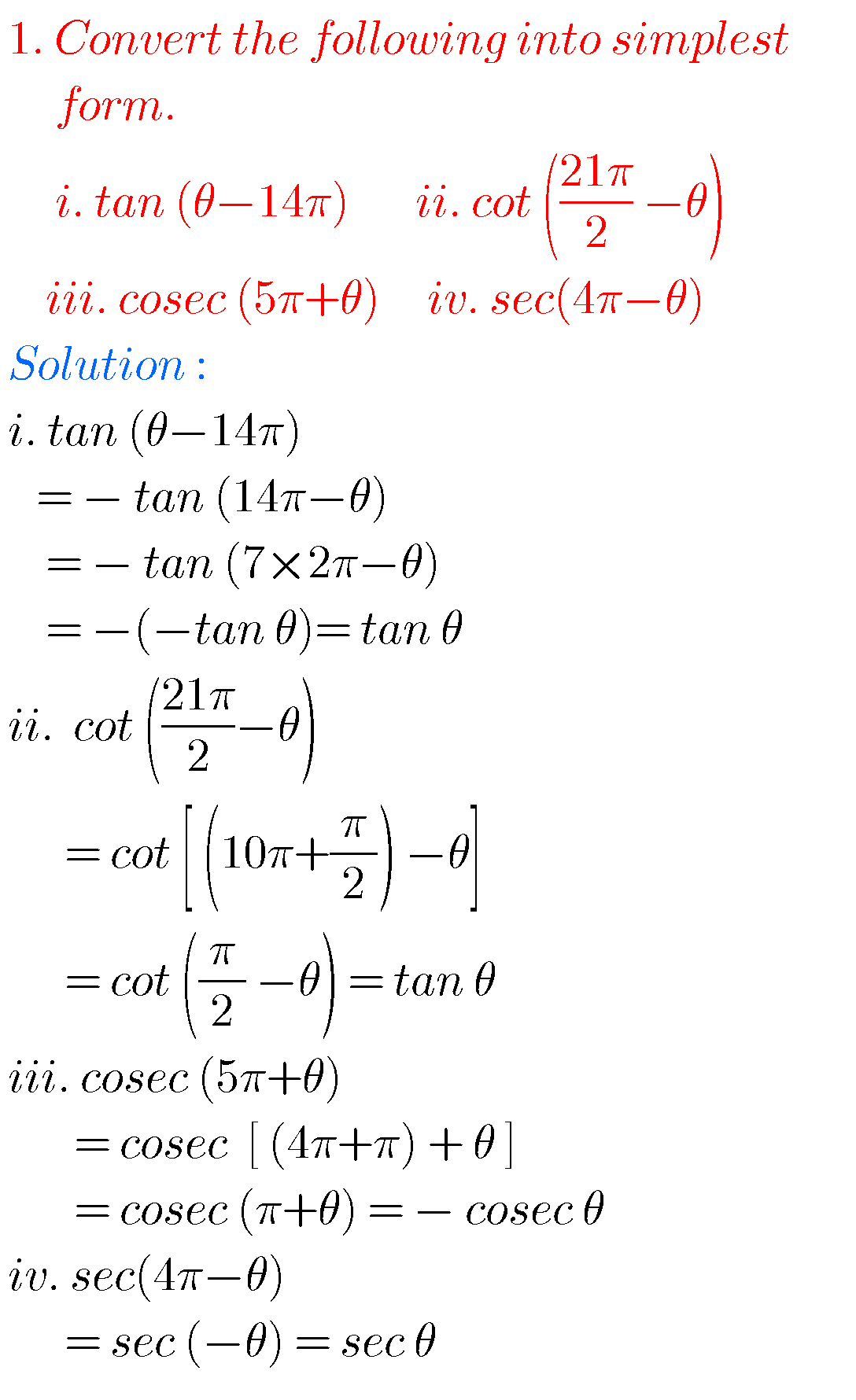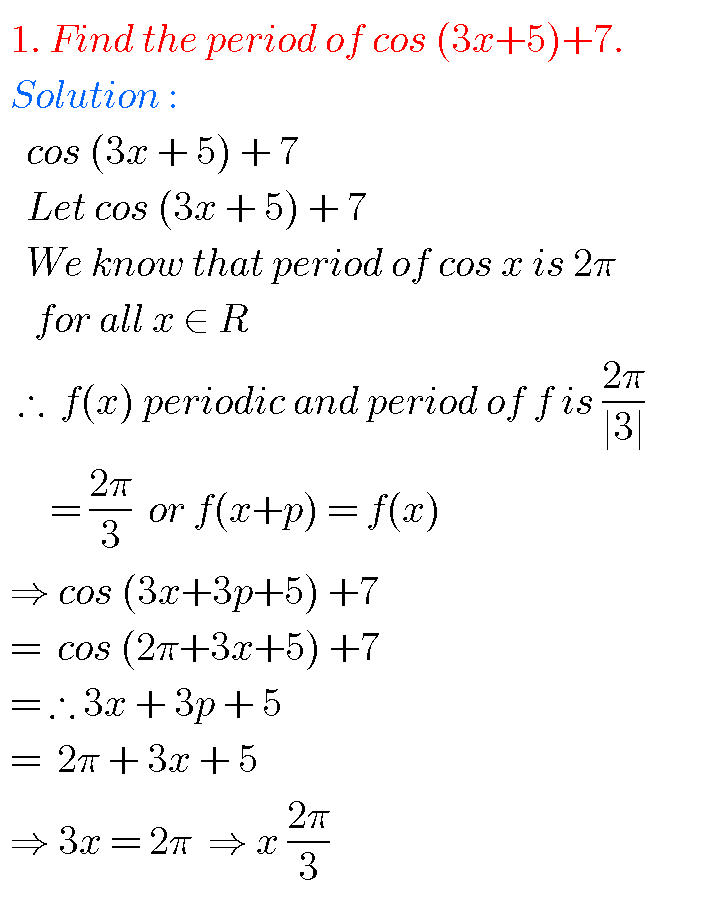Very short answer questions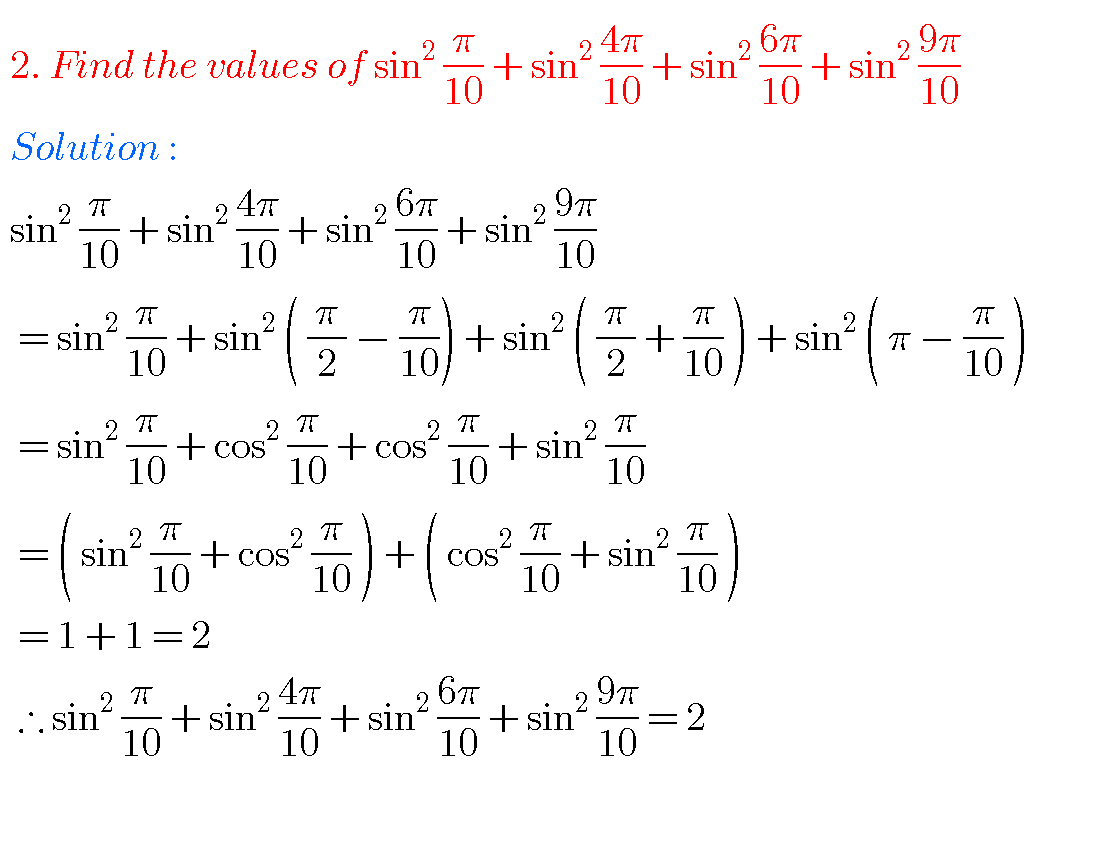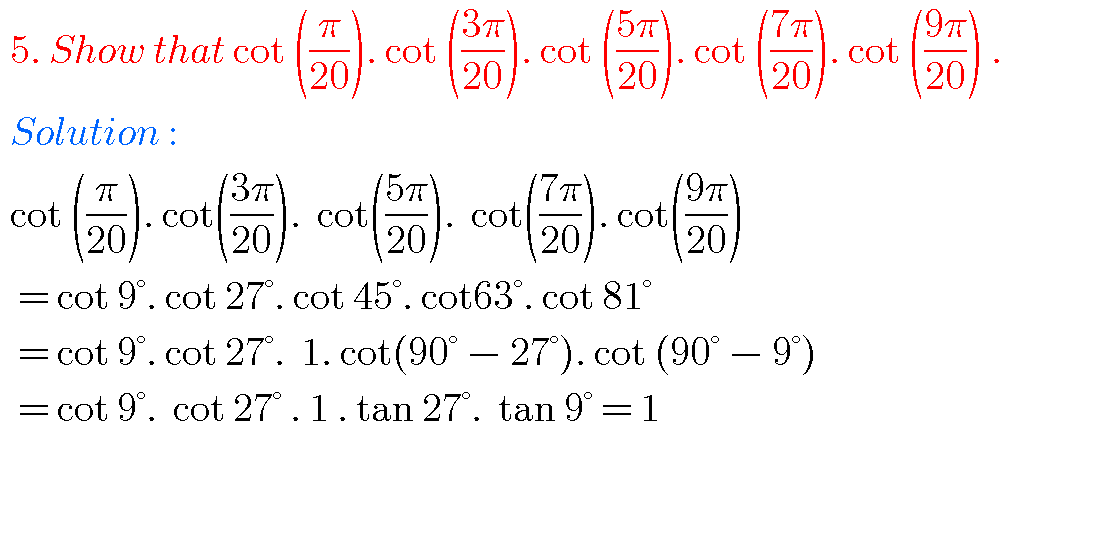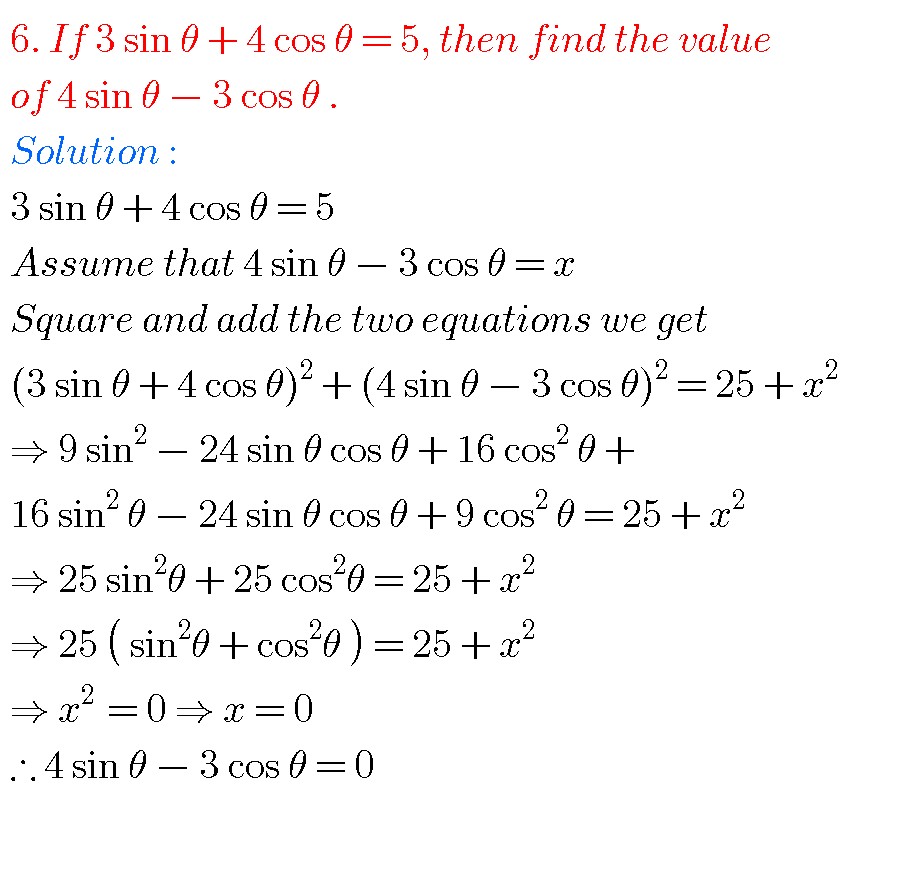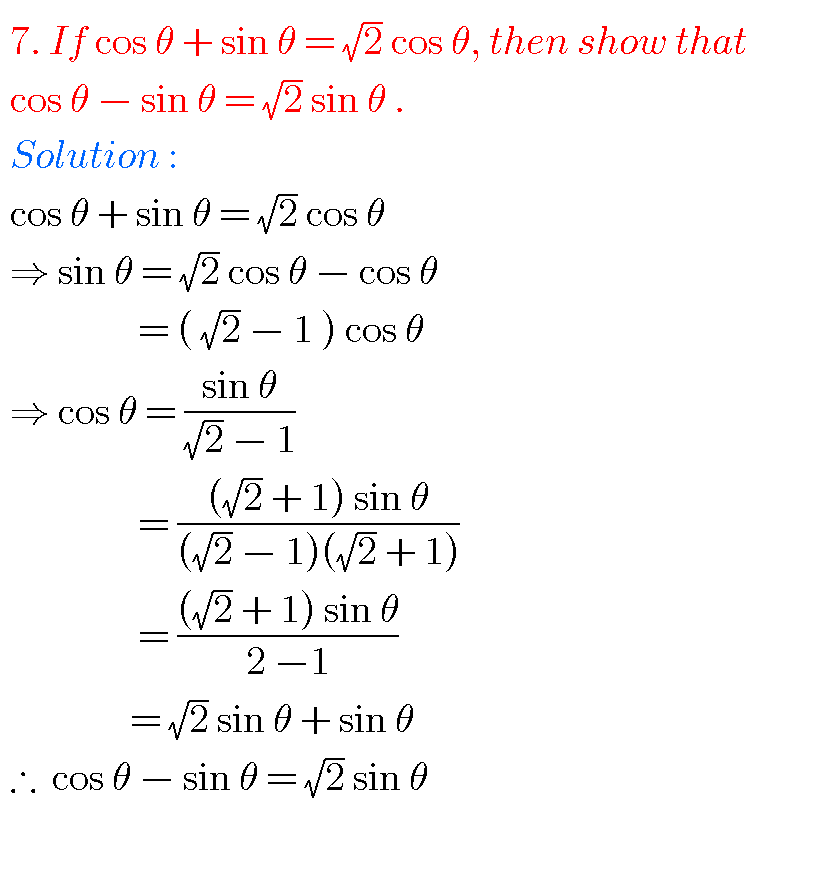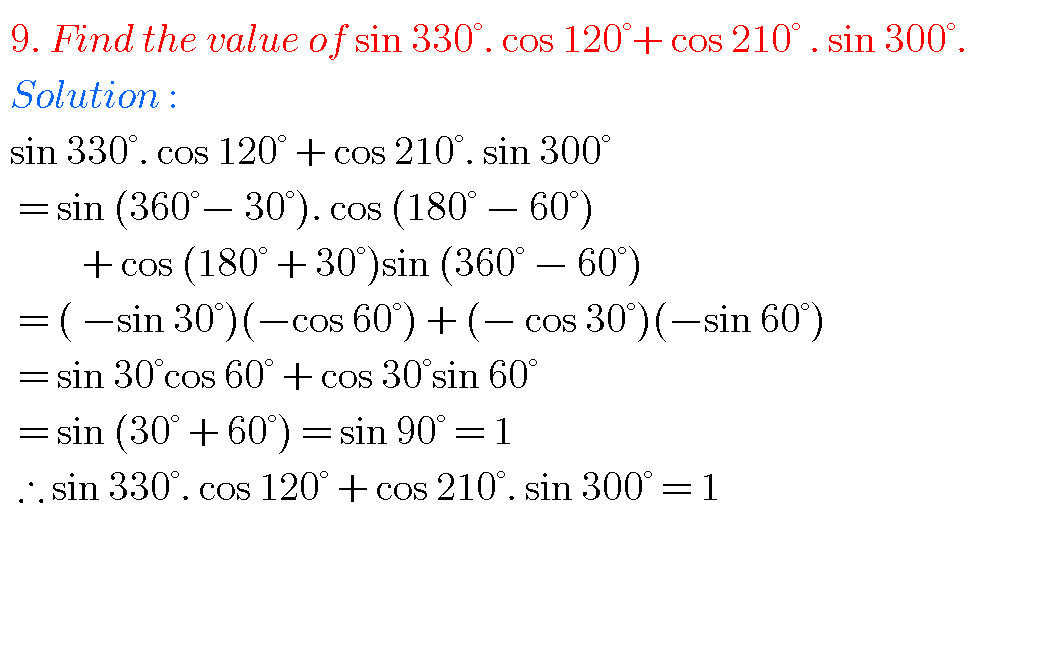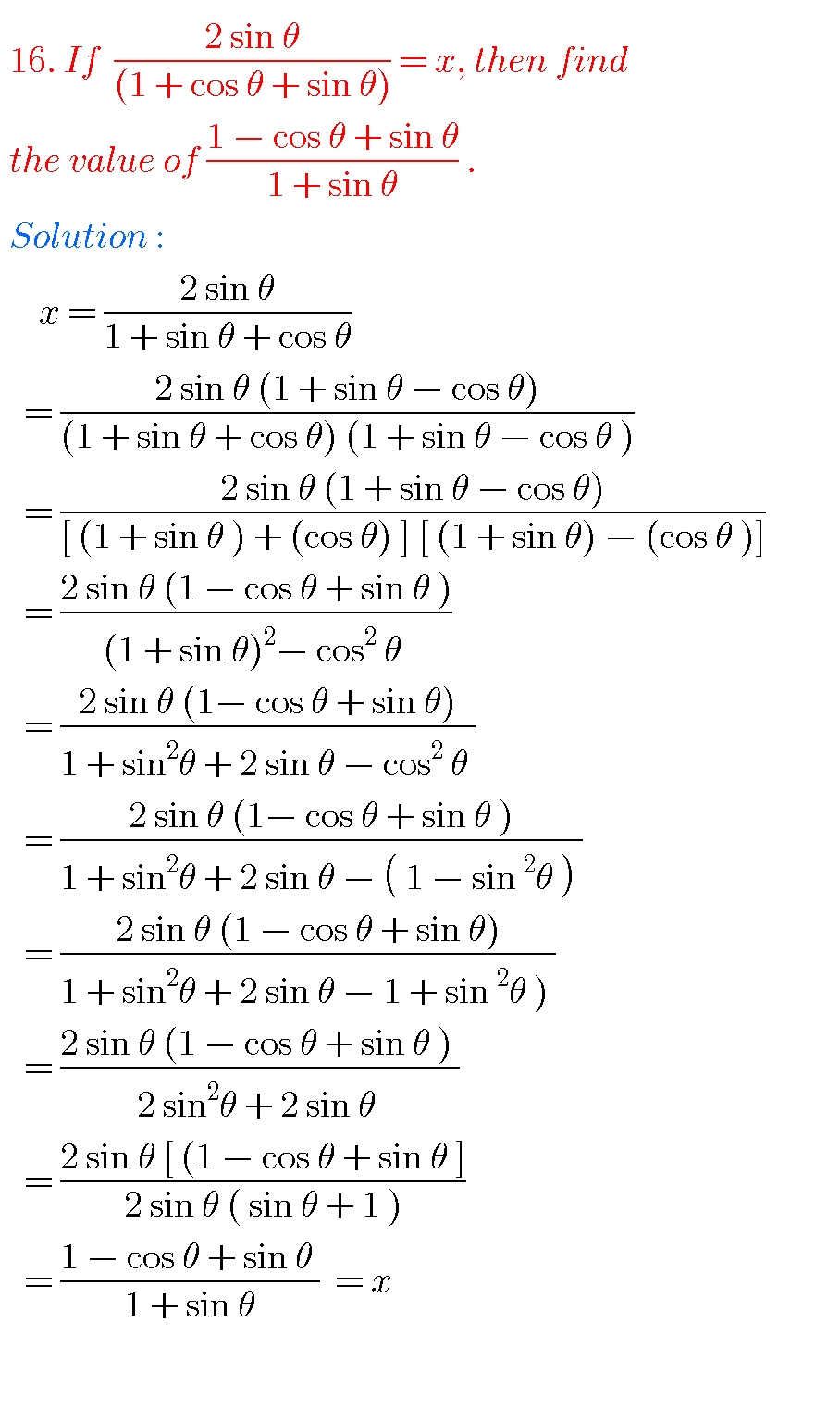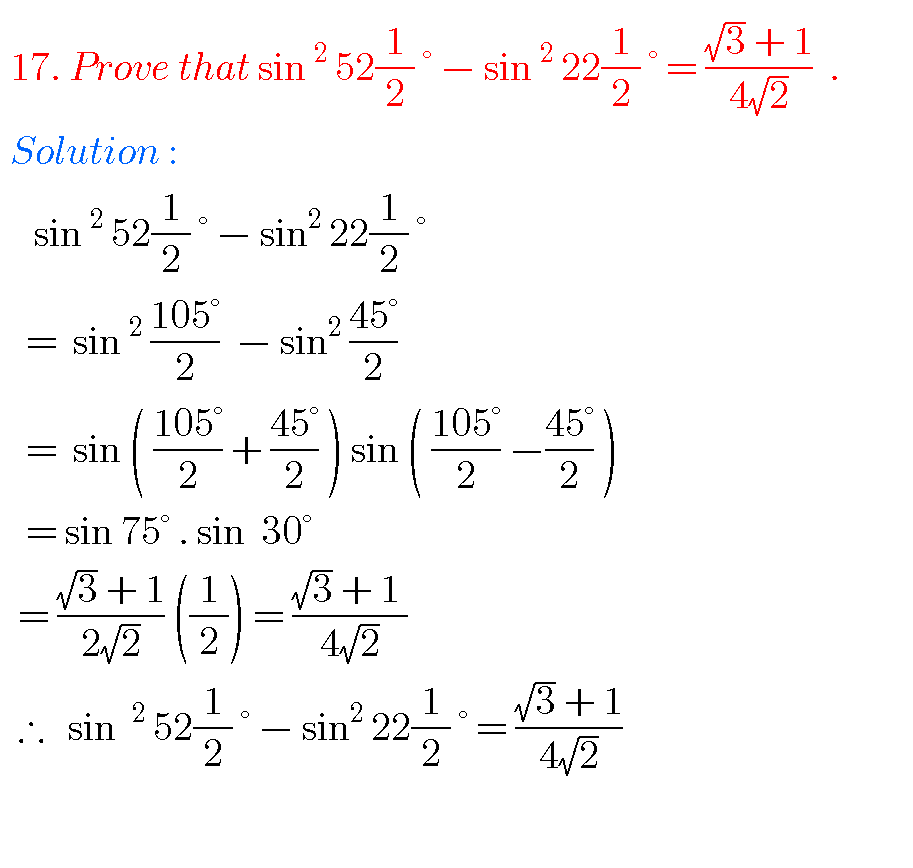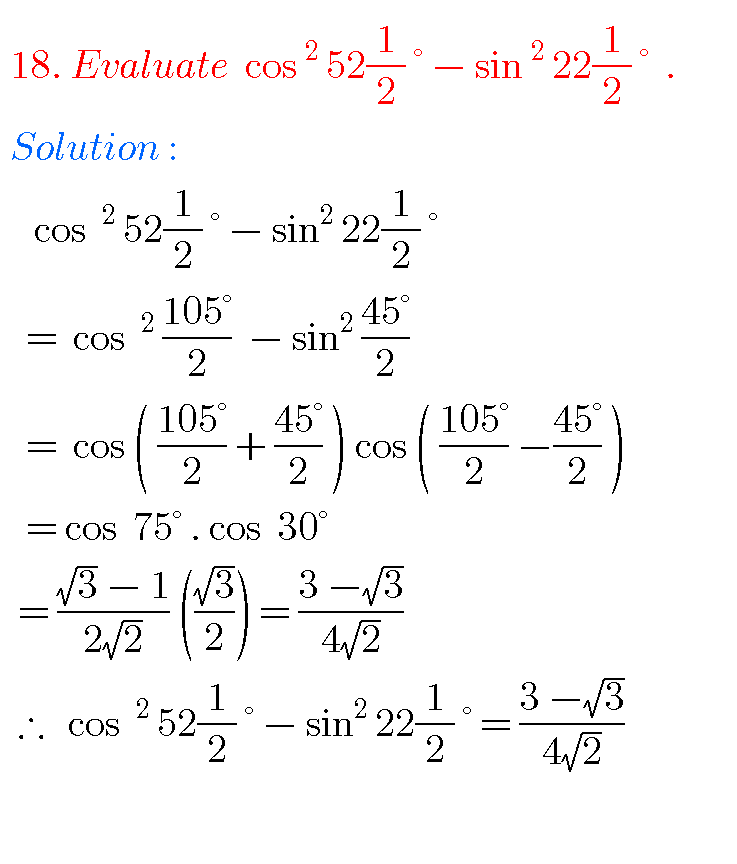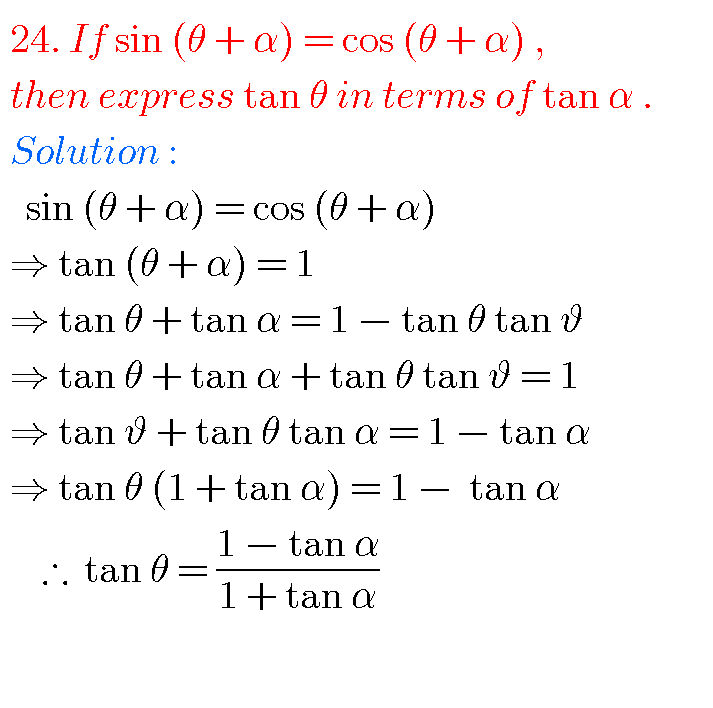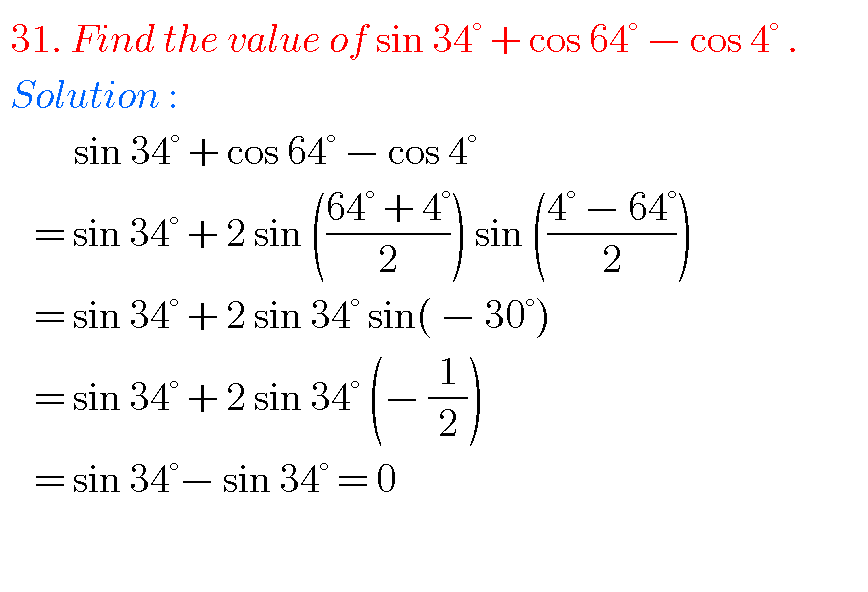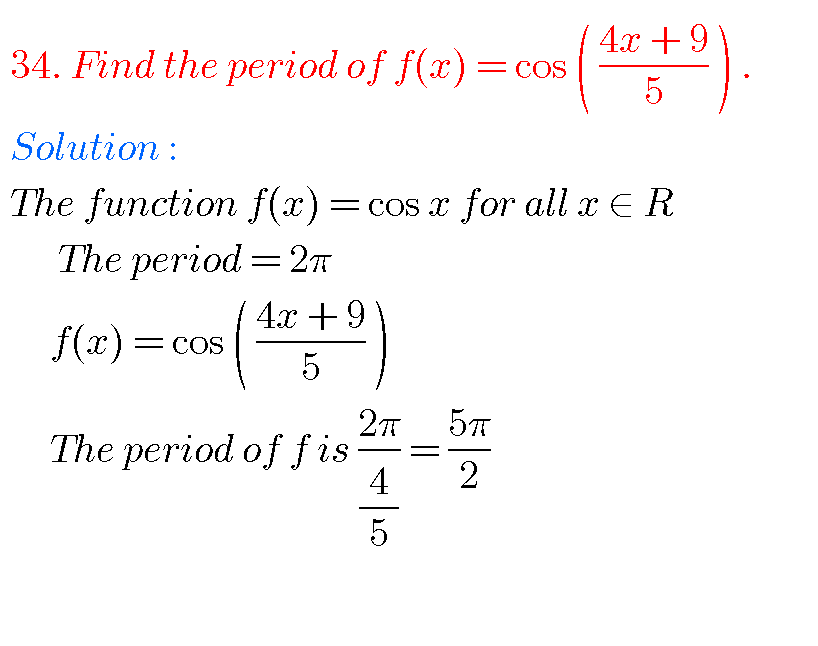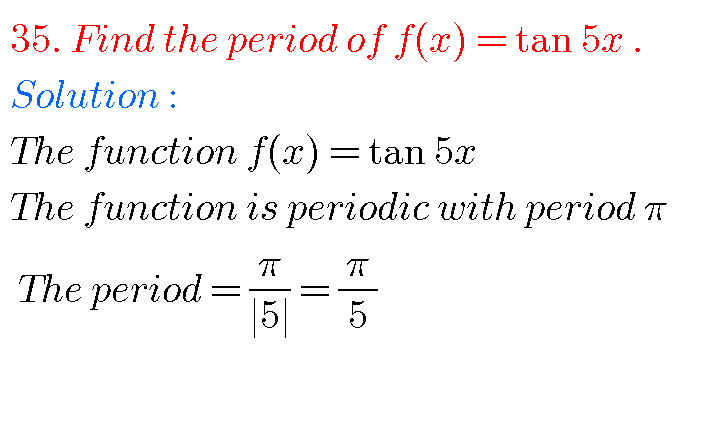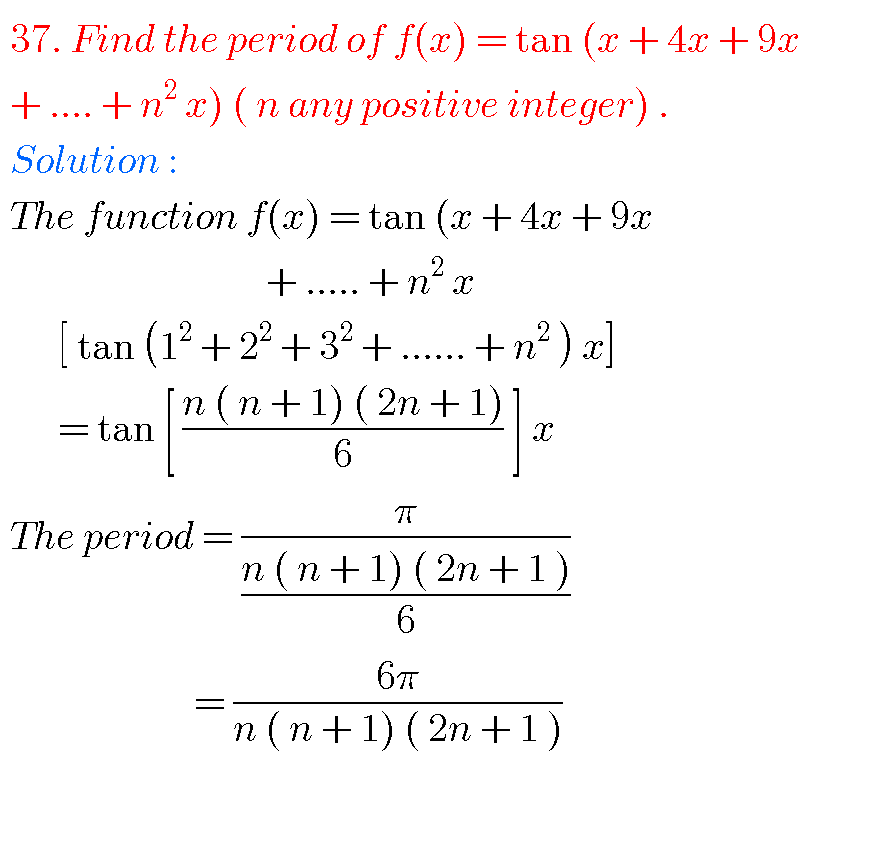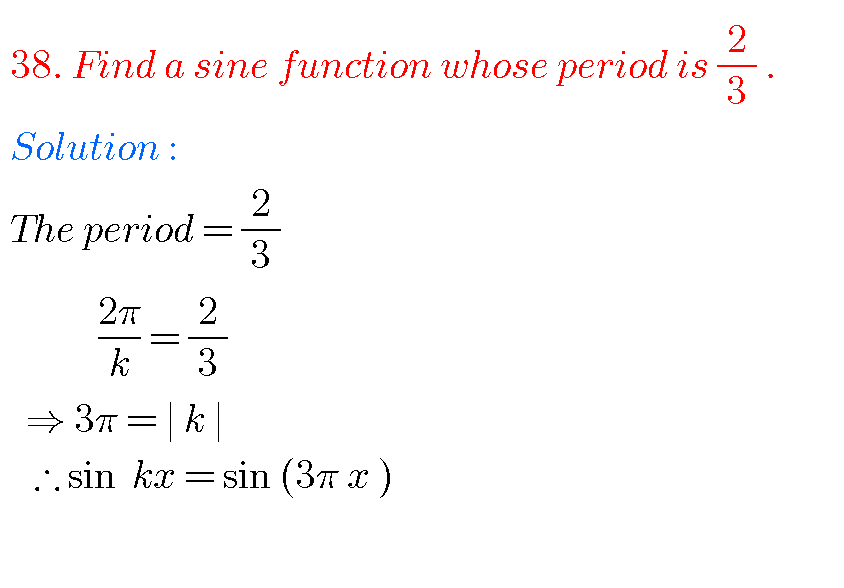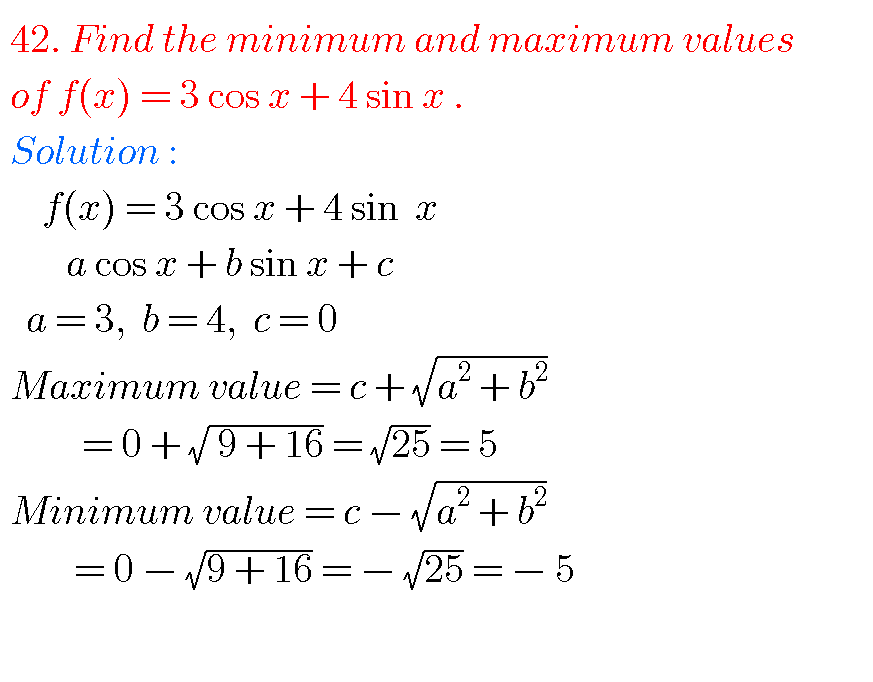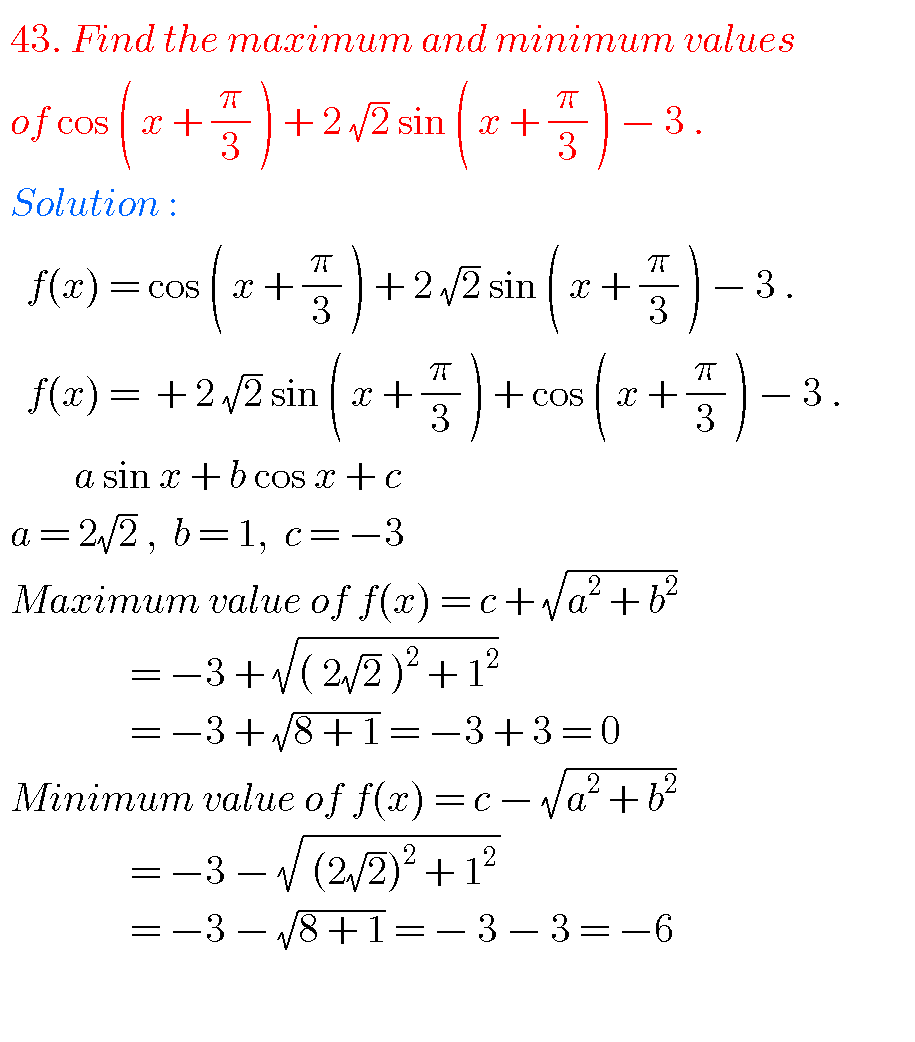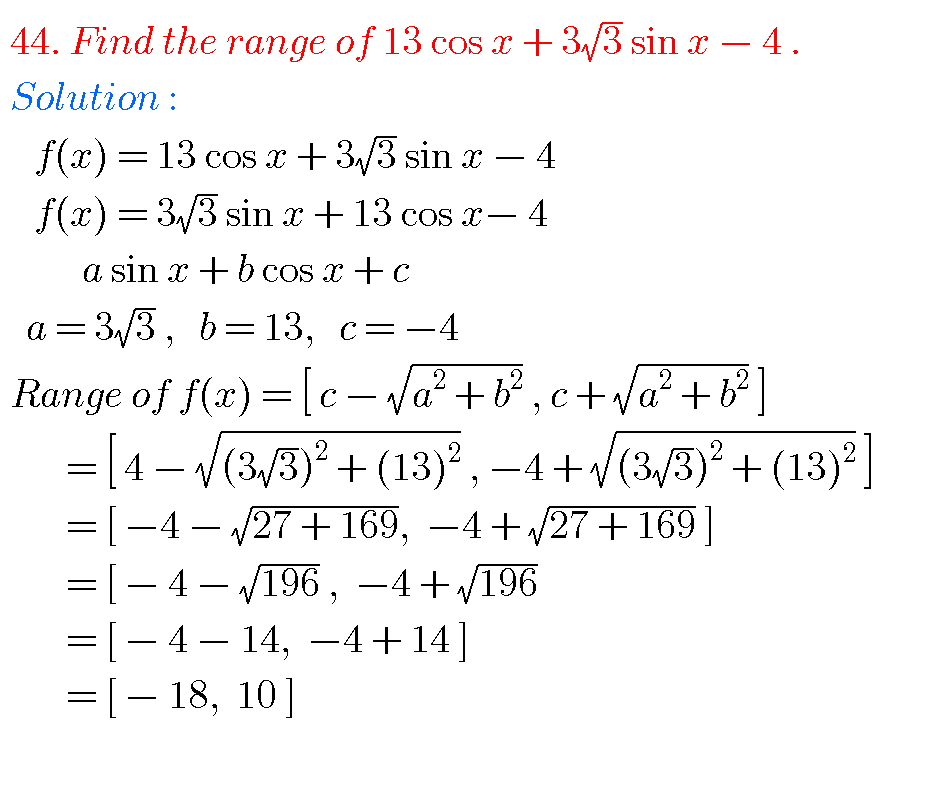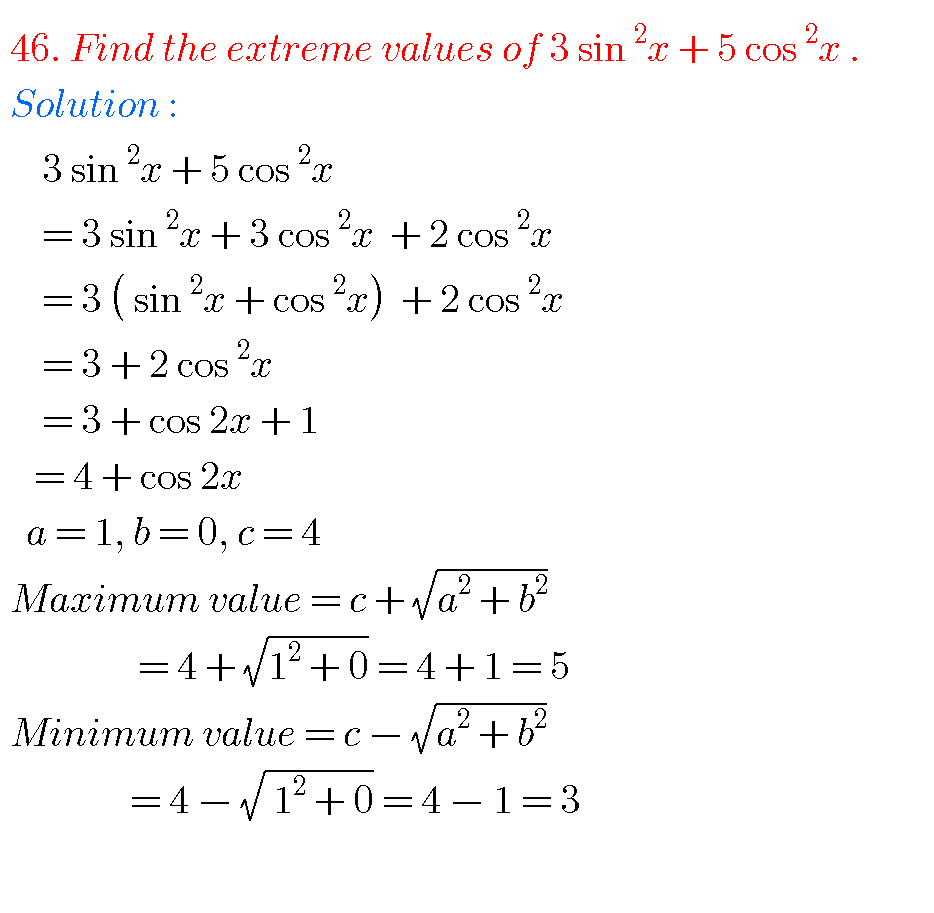## TRIGONOMETRIC RATIOS UPTO TRANSFORMATION, INTERMEDIATE, FIRST YEAR.

Note : Observe the solutions of trigonometric ratios upto transformations and try them in your own methods.

Ncert solutions for maths class 6 th some chapters

Ncert solutions for maths class 7 th some chapters

Ncert solutions for maths class 8 th some chapters

Ncert solutions for class 8 chapter 12

Inter maths 1a solutions

Inter maths trigonometry solutions

Maths real numbers solutions class 10

You can see solutions for Inter Maths IIB

1. Circle

3. Parabola

4. Ellipse

You can also see solutions for Inter Maths IIA

For examination purpose you can see

Complex numbers

De Moivre’ s Theorem

1.G.teja July 17, 2018 at 7:08 pm - Reply

Please put the public examination problems

•Anusha March 17, 2019 at 7:11 pm - Reply

Super

2.Kalyan December 10, 2018 at 6:29 pm - Reply

Thanks you clarifyed my doubts

•Lavanya September 15, 2019 at 1:47 pm - Reply

We need some more problems

3.Madhan July 6, 2019 at 7:30 am - Reply

Plz send me weekend test papers for sri Chaitnaya

4.Madhan July 6, 2019 at 7:31 am - Reply

1year

5.S August 17, 2019 at 3:50 pm - Reply

Try to upload intermediate 1st year maths 1a ch6 exercise problems all …………….. ………

6.Aditya September 18, 2019 at 7:43 pm - Reply

Easy method to understand